OP1 and Bott Periodicity Next: OP1 and Lorentzian Geometry Up: Octonionic Projective Geometry Previous: Projective Lines

## 3.2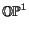and Bott Periodicity

We already touched upon Bott periodicity when we mentioned that the Clifford algebra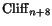is isomorphic to the algebra of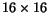matrices with entries lying in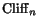. This is but one of many related 'period-8' phenomena that go by the name of Bott periodicity. The appearance of the number 8 here is no coincidence: all these phenomena are related to the octonions! Since this marvelous fact is somewhat under-appreciated, it seems worthwhile to say a bit about it. Here we shall focus on those aspects that are related toand the canonical octonionic line bundle over this space.

Let us start with K-theory. This is a way of gaining information about a topological space by studying the vector bundles over it. If the space has holes in it, there will be nontrivial vector bundles that have 'twists' as we go around these holes. The simplest example is the 'Möbius strip' bundle over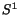, a 1-dimensional real vector bundle which has a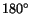twist as we go around the circle. In fact, this is just the canonical line bundle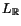. The canonical line bundles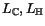and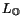provide higher-dimensional analogues of this example.

K-theory tells us to study the vector bundles over a topological space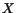by constructing an abelian group as follows. First, take the set consisting of all isomorphism classes of real vector bundles over. Our ability to take direct sums of vector bundles gives this set an 'addition' operation making it into a commutative monoid. Next, adjoin formal 'additive inverses' for all the elements of this set, obtaining an abelian group. This group is called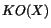, the real K-theory of. Alternatively we could start with complex vector bundles and get a group called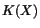, but here we will be interested in real vector bundles.

Any real vector bundle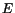overgives an element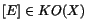, and these elements generate this group. If we pick a point in, there is an obvious homomorphism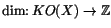sending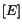to the dimension of the fiber ofat this point. Since the dimension is a rather obvious and boring invariant of vector bundles, it is nice to work with the kernel of this homomorphism, which is called the reduced real K-theory ofand denoted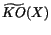. This is an invariant of pointed spaces, i.e. spaces equipped with a designated point or basepoint.

Any sphere becomes a pointed space if we take the north pole as basepoint. The reduced real K-theory of the first eight spheres looks like this: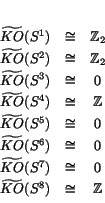where, as one might guess,
•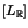generates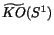.
•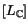generates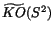.
•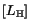generates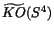.
•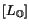generates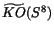.
As mentioned in the previous section, one can build any-dimensional real vector bundle over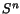using a map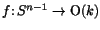. In fact, isomorphism classes of such bundles are in one-to-one correspondence with homotopy classes of such maps. Moreover, two such bundles determine the same element ofif and only if the corresponding maps become homotopy equivalent after we compose them with the inclusion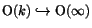, where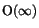is the direct limit of the groups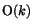. It follows that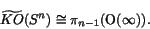This fact gives us the list of homotopy groups ofwhich appears in the Introduction. It also means that to prove Bott periodicity for these homotopy groups: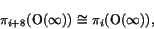it suffices to prove Bott periodicity for real K-theory: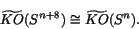Why do we have Bott periodicity in real K-theory? It turns out that there is a graded ring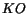with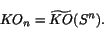The product in this ring comes from our ability to take 'smash products' of spheres and also of real vector bundles over these spheres. Multiplying bygives an isomorphism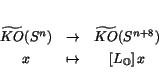In other words, the canonical octonionic line bundle overgenerates Bott periodicity!

There is much more to say about this fact and how it relates to Bott periodicity for Clifford algebras, but alas, this would take us too far afield. We recommend that the interested reader turn to some introductory texts on K-theory, for example the one by Dale Husemoller . Unfortunately, all the books I know downplay the role of the octonions. To spot it, one must bear in mind the relation between the octonions and Clifford algebras, discussed in Section 2.3 above.

Next: OP1 and Lorentzian Geometry Up: Octonionic Projective Geometry Previous: Projective Lines